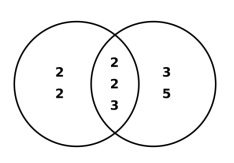# Print N lines of numbers such that every pair among numbers has a GCD K

CServer Side ProgrammingProgramming

## GCD

GCD stands for Greatest Common Divisor of two or more integers excluding 0

Like, to find the greatest common divisor of 48 and 180

48 = 2 × 2 × 2 × 2 × 3

180 = 2 × 2 × 3 × 3 × 5Greatest common divisor = 2 × 2 × 3 = 12.

In the given problem, N lines should be printed with elements have GCD as specified

Input : N=2 GCD=2
Ouput : 2-4-6-10
14-16-18-22

## Algorithm

START
Step 1 -> take input n(e.g. 2) and k(e.g. 2) as int values and i
Step 2-> Loop For i to 0 and i<n and i++
Print (k * (6 * i + 1))
Print (k * (6 * i + 2))
Print (k * (6 * i +3))
Print (k * (6 * i + 5))
Print \n
Step 3 -> end loop
STOP

## Example

#include<stdio.h>
int main() {
int i,n = 2, k = 2;
for (i = 0; i < n; i++) {
printf("%d-",(k * (6 * i + 1)));
printf("%d-",(k * (6 * i + 2)));
printf("%d-",(k * (6 * i + 3)));
printf("%d",(k * (6 * i + 5)));
printf("\n");
}
return 0;
}

## Output

if we run above program then it will generate following output.

2-4-6-10
14-16-18-22